# Python – A Real Contender in the Big Data Arena?!¶

Dr. Yves J. Hilpisch

The Python Quants GmbH

www.pythonquants.com

yves@pythonquants.com

@dyjh

Telefonica, Madrid – 12. February 2014

A brief bio:

• Managing Partner of The Python Quants
• Founder of Visixion GmbH – The Python Quants
• Lecturer Mathematical Finance at Saarland University
• Focus on Financial Industry and Financial Analytics
• Book (2013) "Derivatives Analytics with Python"
• Book (July 2014) "Python for Finance", O'Reilly
• Dr.rer.pol in Mathematical Finance
• Martial Arts Practitioner and Fan

## Python for Analytics¶

Corporations, decision makers and analysts nowadays generally face a number of problems with data:

• sources: data typically comes from different sources, like from the Web, from in-house databases or it is generated in-memory, e.g. for simulation purposes
• formats: data is generally available in different formats, like SQL databases/tables, Excel files, CSV files, arrays, proprietary formats
• structure: data typically comes differently structured, be it unstructured, simply indexed, hierarchically indexed, in table form, in matrix form, in multidimensional arrays
• completeness: real-world data is generally incomplete, i.e. there is missing data (e.g. along an index) or multiple series of data cannot be aligned correctly (e.g. two time series with different time indexes)
• conventions: for some types of data there a many “competing” conventions with regard to formatting, like for dates and time
• interpretation: some data sets contain information that can be easily and intelligently interpreted, like a time index, others not, like texts
• performance: reading, streamlining, aligning, analyzing – i.e. processing – (big) data sets might be slow

In addition to these data-oriented problems, there typically are organizational issues that have to be considered:

• departments: the majority of companies is organized in departments with different technologies, databases, etc., leading to “data silos”
• analytics skills: analytical and business skills in general are possessed by people working in line functions (e.g. production) or administrative functions (e.g. finance)
• technical skills: technical skills, like retrieving data from databases and visualizing them, are generally possessed by people in technology functions (e.g. development, systems operations)

This presentation focuses on

• Python as a general purpose data analytics environment
• interactive analytics examples
• prototyping-like Python usage

It does not address such important issues like

• architectural issues regarding hardware and software
• development processes, testing, documentation and production
• real world problem modeling

## Some Python Fundamentals¶

### Fundamental Python Libraries¶

A fundamental Python stack for interactive data analytics and visualization should at least contain the following libraries tools:

• Python – the Python interpreter itself
• NumPy – high performance, flexible array structures and operations
• SciPy – collection of scientific modules and functions (e.g. for regression, optimization, integration)
• pandas – time series and panel data analysis and I/O
• PyTables – hierarchical, high performance database (e.g. for out-of-memory analytics)
• matplotlib – 2d and 3d visualization
• IPython – interactive data analytics, visualization, publishing

It is best to use either the Python distribution Anaconda or the Web-based analytics environment Wakari. Both provide almost "complete" Python environments.

For example, pandas can, among others, help with the following data-related problems:

• sources: pandas reads data directly from different data sources such as SQL databases, Excel files or JSON based APIs
• formats: pandas can process input data in different formats like CSV files or Excel files; it can also generate output in different formats like CSV, XLS, HTML or JSON
• structure: pandas' strength lies in structured data formats, like time series and panel data
• completeness: pandas automatically deals with missing data in most circumstances, e.g. computing sums even if there are a few or many “not a number”, i.e. missing, values
• conventions/interpretation: for example, pandas can interpret and convert different date-time formats to Python datetime objects and/or timestamps
• performance: the majority of pandas classes, methods and functions is implemented in a performance-oriented fashion making heavy use of the Python/C compiler Cython

### First Analytics Steps with Python¶

As a simple example let's generate a NumPy array with five sets of 1000 (pseudo-)random numbers each.

In :
import numpy as np  # this imports the NumPy library

In :
data = np.random.standard_normal((5, 1000))  # generate 5 sets with 1000 rn each
data[:, :5].round(3)  # print first five values of each set rounded to 3 digits

Out:
array([[ 0.047,  0.056, -0.985, -0.347,  1.179],
[-0.802, -0.009, -0.365,  0.866, -1.323],
[ 0.594,  1.06 , -1.192,  1.286,  2.054],
[-0.229, -0.932,  0.808,  0.579, -0.53 ],
[ 1.466,  0.757, -0.627,  0.321,  0.396]])


Let's plot a histogram of the 1st, 2nd and 3rd data set.

In :
import matplotlib.pyplot as plt  # this imports the plotting library
%matplotlib inline
# turn on inline plotting

In :
plt.hist([data, data, data], label=['Set 0', 'Set 1', 'Set 2'])
plt.grid(True)  # grid for better readability
plt.legend()

Out:
<matplotlib.legend.Legend at 0x2ed6650>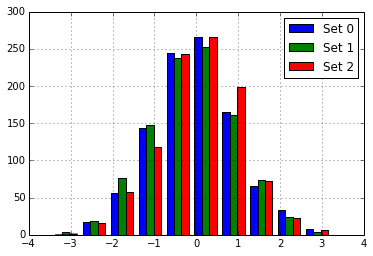We then want to plot the (running) cumulative sum of each set.

In :
plt.figure()  # initialize figure object
plt.grid(True)
for data_set in enumerate(data):  # iterate over all rows
plt.plot(data_set.cumsum(), label='Set %s' % data_set)
# plot the running cumulative sums for each row
plt.legend(loc=0)  # write legend with labels

Out:
<matplotlib.legend.Legend at 0x30dba90>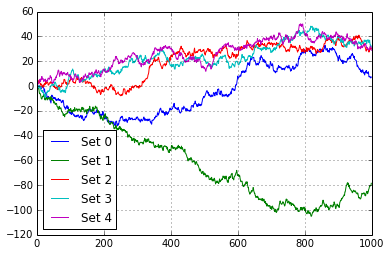Some fundamental statistics from our data sets.

In :
data.mean(axis=1)  # average value of the 5 sets

Out:
array([ 0.00719361, -0.07845145,  0.03071323,  0.0314976 ,  0.02877251])

In :
data.std(axis=1)  # standard deviation of the 5 sets

Out:
array([ 0.99718217,  1.02842477,  0.97160148,  1.001481  ,  1.00440801])

In :
np.round(np.corrcoef(data), 2)  # correlation matrix of the 5 data sets

Out:
array([[ 1.  , -0.03, -0.05, -0.02,  0.02],
[-0.03,  1.  , -0.04,  0.03, -0.1 ],
[-0.05, -0.04,  1.  , -0.  ,  0.01],
[-0.02,  0.03, -0.  ,  1.  ,  0.06],
[ 0.02, -0.1 ,  0.01,  0.06,  1.  ]])


## Efficient Data Analytics with Python, pandas & Co.¶

### Creating a Database to Work With¶

Currently, and in the foreseeable future as well, most corporate data is stored in SQL databases. We take this as a starting point and generate a SQL table with dummy data.

In :
filename = './data/numbers'
import sqlite3 as sq3  # imports the SQLite library


We create a table and populate the table with the dummy data.

In :
query = 'CREATE TABLE numbs (no1 real, no2 real, no3 real, no4 real, no5 real)'
con = sq3.connect(filename + '.db')
con.execute(query)
con.commit()

In :
# Writing Random Numbers to SQLite3 Database
rows = 2000000  # 2,000,000 rows for the SQL table (ca. 100 MB in size)
nr = np.random.standard_normal((rows, 5))
%time con.executemany('INSERT INTO numbs VALUES(?, ?, ?, ?, ?)', nr)
con.commit()

CPU times: user 29.7 s, sys: 507 ms, total: 30.2 s
Wall time: 31.4 s



### Reading Data into NumPy Arrays¶

We can now read the data into a NumPy array.

In :
# Reading a couple of rows from SQLite3 database table
np.array(con.execute('SELECT * FROM numbs').fetchmany(3)).round(3)

Out:
array([[-0.849, -1.22 ,  0.236,  0.077,  0.351],
[-0.469, -2.048, -1.467, -1.955,  0.413],
[ 1.558,  0.841,  0.508,  1.502,  0.706]])

In :
# Reading the whole table into an NumPy array
%time result = np.array(con.execute('SELECT * FROM numbs').fetchall())

CPU times: user 11.6 s, sys: 276 ms, total: 11.9 s
Wall time: 11.9 s


In :
result[:3].round(3)  # the same rows from the in-memory array

Out:
array([[-0.849, -1.22 ,  0.236,  0.077,  0.351],
[-0.469, -2.048, -1.467, -1.955,  0.413],
[ 1.558,  0.841,  0.508,  1.502,  0.706]])

In :
nr = 0.0; result = 0.0  # memory clean-up


pandas is optimized both for convenience and performance when it comes to analytics and I/O operations.

In :
import pandas as pd
import pandas.io.sql as pds

In :
%time data = pds.read_frame('SELECT * FROM numbs', con)
con.close()

CPU times: user 4.1 s, sys: 282 ms, total: 4.38 s
Wall time: 4.38 s


In :
data.head()

Out:
no1 no2 no3 no4 no5
0 -0.848849 -1.219614 0.236473 0.076592 0.351047
1 -0.469214 -2.048383 -1.466683 -1.954855 0.413186
2 1.557722 0.840650 0.507778 1.501894 0.706384
3 0.884471 -0.005464 0.006768 -0.668019 -1.596972
4 0.065458 0.942663 -0.623747 -1.187146 2.751866

The plotting of larger data sets often is a problem. This can easily be accomplished here by only plotting every 500th data row, for instance.

In :
data[::500].cumsum().plot(grid=True, legend=True)  # every 500th row is plotted

Out:
<matplotlib.axes.AxesSubplot at 0x3ee0d90>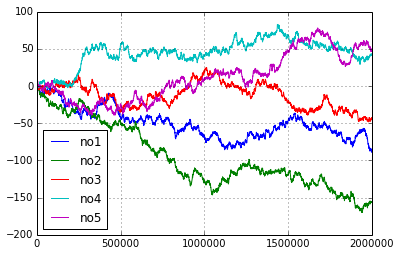### Writing Data with pandas¶

There are some reasons why you may want to write the DataFrame to disk:

• Database Performance: you do not want to query the SQL database everytime anew
• IO Speed: you want to increase data/information retrievel
• Data Structure: maybe you want to store additional data with the original data set or you have generated a DataFrame out of multiple sources

pandas is tightly integrated with PyTables, a library that implements the HDF5 standard for Python. It makes data storage and I/O operations not only convenient but quite fast as well.

In :
h5 = pd.HDFStore(filename + '.h5', 'w')
%time h5['data'] = data  # writes the DataFrame to HDF5 database

CPU times: user 71 ms, sys: 109 ms, total: 180 ms
Wall time: 431 ms



Now, we can read the data from the HDF5 file into another DataFrame object. Performance of such an operation generally is quite high.

In :
%time data_new = h5['data']

CPU times: user 4 ms, sys: 29 ms, total: 33 ms
Wall time: 33.2 ms


In :
%time data.sum() - data_new.sum()   # checking if the DataFrames are the same

CPU times: user 294 ms, sys: 1 ms, total: 295 ms
Wall time: 295 ms


Out:
no1    0
no2    0
no3    0
no4    0
no5    0
dtype: float64

In :
%time np.allclose(data, data_new, rtol=0.1)

CPU times: user 497 ms, sys: 29 ms, total: 526 ms
Wall time: 525 ms


Out:
True

In :
data_new = 0.0  # memory clean-up
h5.close()


### In-Memory Analytics with pandas¶

pandas has powerful selection and querying mechanisms (I).

In :
res = data[['no1', 'no2']][((data['no2'] > 0.5) | (data['no2'] < -0.5))]

In :
plt.plot(res.no1, res.no2, 'ro')
plt.grid(True); plt.axis('tight')

Out:
(-4.6341840011538578,
4.7603360452756149,
-4.868748829943951,
5.0798716055278774)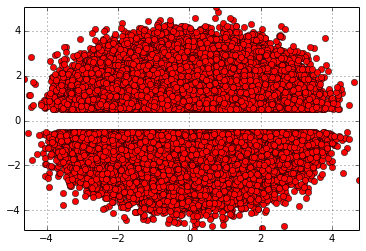pandas has powerful selection and querying mechanisms (II).

In :
res = data[['no1', 'no2']][((data['no1'] > 0.5) | (data['no1'] < -0.5))
& ((data['no2'] < -1) | (data['no2'] > 1))]

In :
plt.plot(res.no1, res.no2, 'ro')
plt.grid(True); plt.axis('tight')

Out:
(-4.6341840011538578,
4.7603360452756149,
-4.7750688381858097,
5.0798716055278774)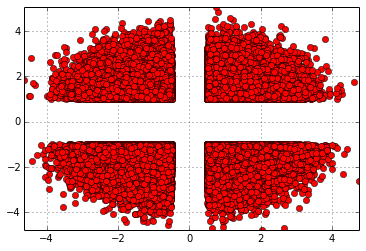pandas has powerful selection and querying mechanisms (III).

In :
res = data[['no1', 'no2', 'no3' ]][((data['no1'] > 1.5) | (data['no1'] < -1.5))
& ((data['no2'] < -1) | (data['no2'] > 1))
& ((data['no3'] < -1) | (data['no3'] > 1))]

In :
from mpl_toolkits.mplot3d import Axes3D
fig = plt.figure(figsize=(8, 5))
ax.scatter(res.no1, res.no2, res.no3, zdir='z', s=25, c='r', marker='o')
ax.set_xlabel('no1'); ax.set_ylabel('no2'); ax.set_zlabel('no3')

Out:
<matplotlib.text.Text at 0x451fe10>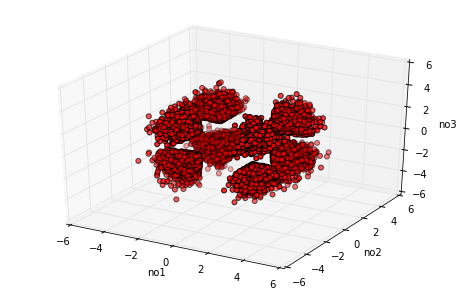### Exporting Data with pandas¶

The data is easily exported to different standard data file formats like comma separated value files (CSV).

In :
%time data.to_csv(filename + '.csv')  # writes the whole data set as CSV file

CPU times: user 19.9 s, sys: 465 ms, total: 20.3 s
Wall time: 20.9 s


In :
csv_file = open(filename + '.csv')
for i in range(5):

,no1,no2,no3,no4,no5
0,-0.8488490114302236,-1.219614432339102,0.23647266112406093,0.07659168219031565,0.35104692376922414
1,-0.4692140821675998,-2.0483834921562925,-1.466682965538265,-1.9548546546734777,0.41318639065284635
2,1.5577216710707784,0.840649586202206,0.5077783468072868,1.501894262199288,0.706384462326364
3,0.8844706403478411,-0.005464013593834427,0.006768134738374346,-0.6680186262598709,-1.5969723088232077



pandas also interacts easily with Microsoft Excel spreadsheet files (XLS/XLSX).

In :
%time data.ix[:10000].to_excel(filename + '.xlsx')  # first 10,000 rows written in a Excel file

CPU times: user 21.7 s, sys: 28 ms, total: 21.7 s
Wall time: 21.7 s



With pandas you can in one step read data from an Excel file and e.g. plot it.

In :
%time pd.read_excel(filename + '.xlsx', 'sheet1').hist(grid=True)

CPU times: user 1.78 s, sys: 1e+03 µs, total: 1.78 s
Wall time: 1.78 s


Out:
array([[Axes(0.125,0.563043;0.215278x0.336957),
Axes(0.404861,0.563043;0.215278x0.336957),
Axes(0.684722,0.563043;0.215278x0.336957)],
[Axes(0.125,0.125;0.215278x0.336957),
Axes(0.404861,0.125;0.215278x0.336957),
Axes(0.684722,0.125;0.215278x0.336957)]], dtype=object)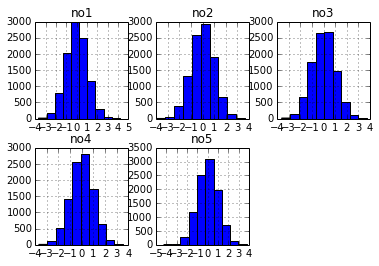One can also generate HTML code, e.g. for automatic Web-based report generation.

In :
html_code = data.ix[:2].to_html()
html_code

Out:
u'<table border="1" class="dataframe">\n  <thead>\n    <tr style="text-align: right;">\n      <th></th>\n      <th>no1</th>\n      <th>no2</th>\n      <th>no3</th>\n      <th>no4</th>\n      <th>no5</th>\n    </tr>\n  </thead>\n  <tbody>\n    <tr>\n      <th>0</th>\n      <td>-0.848849</td>\n      <td>-1.219614</td>\n      <td> 0.236473</td>\n      <td> 0.076592</td>\n      <td> 0.351047</td>\n    </tr>\n    <tr>\n      <th>1</th>\n      <td>-0.469214</td>\n      <td>-2.048383</td>\n      <td>-1.466683</td>\n      <td>-1.954855</td>\n      <td> 0.413186</td>\n    </tr>\n    <tr>\n      <th>2</th>\n      <td> 1.557722</td>\n      <td> 0.840650</td>\n      <td> 0.507778</td>\n      <td> 1.501894</td>\n      <td> 0.706384</td>\n    </tr>\n  </tbody>\n</table>'

In :
from IPython.core.display import HTML

In :
HTML(html_code)

Out:
no1 no2 no3 no4 no5
0 -0.848849 -1.219614 0.236473 0.076592 0.351047
1 -0.469214 -2.048383 -1.466683 -1.954855 0.413186
2 1.557722 0.840650 0.507778 1.501894 0.706384

Some final checks and data cleaning.

In :
ll ./data/numb*

-rw-rw-rw- 1 root 211198950 11. Feb 19:04 ./data/numbers.csv
-rw-r--r-- 1 root 108890112 11. Feb 19:03 ./data/numbers.db
-rw-rw-rw- 1 root  96007368 11. Feb 19:04 ./data/numbers.h5
-rw-rw-rw- 1 root    780373 11. Feb 19:05 ./data/numbers.xlsx


In :
data = 0.0  # memory clean-up


## Hardware Bound IO Operations with SSD Drives¶

### NumPy Array Dummy Data¶

We will operate on arrays and tables with 100,000,000 rows and 5 columns.

In :
import numpy as np

In :
n = 100000000

In :
dt = np.dtype([('no1', 'f8'), ('no2', 'f8'), ('no3', 'f8'),
('no4', 'f8'), ('no5', 'f8')])


With the number of rows n and the data type dt, we can now generate the NumPy array.

In :
%time array = np.array(np.random.randint(low=0, high=10, size=n), dtype=dt)

CPU times: user 4 s, sys: 719 ms, total: 4.72 s
Wall time: 4.73 s


In :
array[:2]

Out:
array([(9.0, 9.0, 9.0, 9.0, 9.0), (5.0, 5.0, 5.0, 5.0, 5.0)],
dtype=[('no1', '<f8'), ('no2', '<f8'), ('no3', '<f8'), ('no4', '<f8'), ('no5', '<f8')])


We have 4GB of data; so far in-memory.

In :
array.nbytes

Out:
4000000000


### Storage in Table – No Compression¶

We first open a HDF5 database and use the PyTables library to this end.

In :
import tables as tb

In :
h5 = tb.open_file('./data/data.h5', 'w')


We then write the NumPy array to a PyTables table object.

In :
%time h5.create_table(where='/', name='table', description=array)

CPU times: user 1.08 s, sys: 4.53 s, total: 5.6 s
Wall time: 6.58 s


Out:
/table (Table(100000000,)) ''
description := {
"no1": Float64Col(shape=(), dflt=0.0, pos=0),
"no2": Float64Col(shape=(), dflt=0.0, pos=1),
"no3": Float64Col(shape=(), dflt=0.0, pos=2),
"no4": Float64Col(shape=(), dflt=0.0, pos=3),
"no5": Float64Col(shape=(), dflt=0.0, pos=4)}
byteorder := 'little'
chunkshape := (13107,)


Writing speed is almost at the limit of the SSD drive, i.e. about 500 MB/s.

In :
h5.close()

In :
ll ./data

insgesamt 3910608
-rw-rw-rw- 1 root 4000550328 11. Feb 19:05 data.h5



We now have the 4GB of data on disk.

### Storage in Table – With Compression¶

A new database object.

In :
h5 = tb.open_file('./data/data_comp.h5', 'w')


Now use compression for the same IO operation.

In :
filters = tb.Filters(complevel=9, complib='blosc', shuffle=True, fletcher32=False)

In :
%time h5.create_table(where='/', name='table', description=array, filters=filters)

CPU times: user 19.4 s, sys: 2.12 s, total: 21.6 s
Wall time: 12.5 s


Out:
/table (Table(100000000,), shuffle, blosc(9)) ''
description := {
"no1": Float64Col(shape=(), dflt=0.0, pos=0),
"no2": Float64Col(shape=(), dflt=0.0, pos=1),
"no3": Float64Col(shape=(), dflt=0.0, pos=2),
"no4": Float64Col(shape=(), dflt=0.0, pos=3),
"no5": Float64Col(shape=(), dflt=0.0, pos=4)}
byteorder := 'little'
chunkshape := (13107,)


Writing with compression takes only a bit longer.

In :
h5.close()

In :
ll ./data

insgesamt 4056320
-rw-rw-rw- 1 root  149056793 11. Feb 19:06 data_comp.h5
-rw-rw-rw- 1 root 4000550328 11. Feb 19:05 data.h5



However, in this particular case the 4GB data set is compressed to about 150MB only. This is a huge advantage, e.g. for backup routines.

## Fast Evaluation of Numerical Expressions¶

### In-Memory Approach¶

The numexpr is specifically designed for the fast evaluation of numerical expressions.

In :
import numexpr as ne


For simplicity, we use another array with random data but with the same size and structure as in the previous example.

In :
array = np.abs(np.random.standard_normal((n, 5)))


All operations to follow will be on 500,000,000 floating point numbers.

In :
array.size

Out:
500000000


The task is to evaluate the following numerical expression:

$y = \sin(x)^2 + \sqrt{x}$

To begin with, we set number of threads to 1 ("single threading").

In :
ne.set_num_threads(1)
%time ex = ne.evaluate('sin(array) ** 2 + sqrt(array)')

CPU times: user 7.86 s, sys: 702 ms, total: 8.56 s
Wall time: 8.57 s


In :
ex[:2]

Out:
array([[ 2.16490918,  1.08196853,  0.30623592,  1.70940274,  0.54777368],
[ 0.77281341,  2.19262513,  1.17907809,  2.27096836,  0.98273457]])


numexpr has built-in multi-threading. Now set number of threads to 16 and evaluate the expression.

In :
ne.set_num_threads(16)
%time ex = ne.evaluate('sin(array) ** 2 + sqrt(array)')

CPU times: user 15 s, sys: 1.04 s, total: 16.1 s
Wall time: 1.07 s



Multi-threading of course brings a lot for such problems.

### Out-of-Memory Approach¶

For the out-of-memory evaluation of the numerical expression, we use again a HDF5 database via PyTables.

In :
h5 = tb.open_file('./data/oomemory.h5', 'w')


We write the array to a PyTables array object on disk.

In :
%time darray = h5.create_array('/', 'array', array)

CPU times: user 0 ns, sys: 4.7 s, total: 4.7 s
Wall time: 5.5 s



For out-of-memory calculations, we also need a results array object on disk.

In :
%time out = h5.create_array('/', 'out', np.zeros_like(array))

CPU times: user 308 ms, sys: 5.53 s, total: 5.84 s
Wall time: 7.8 s


In :
ll ./data

insgesamt 11876468
-rw-rw-rw- 1 root  149056793 11. Feb 19:06 data_comp.h5
-rw-rw-rw- 1 root 4000550328 11. Feb 19:05 data.h5
-rw-rw-rw- 1 root 8000002304 11. Feb 19:07 oomemory.h5



The out-of-memory evaluation of the numerical expression.

In :
%%time
ext = tb.Expr('sin(darray) ** 2 + sqrt(darray)')
ext.setOutput(out)
ext.eval()

CPU times: user 16.9 s, sys: 3.8 s, total: 20.7 s
Wall time: 5.32 s



Check if we got the same results.

In :
out[:2]

Out:
array([[ 2.16490918,  1.08196853,  0.30623592,  1.70940274,  0.54777368],
[ 0.77281341,  2.19262513,  1.17907809,  2.27096836,  0.98273457]])

In :
h5.close()


## Massively Parallel Generation of Random Numbers¶

The use Nvidia GPUs, we need to have CUDA installed. An easy way to harness the power of Nvidia GPUs is the use of Continuum's NumbaPro library that dynamically compiles Python code for the GPU.

In :
from numbapro.cudalib import curand
import time as t


As the benchmark case, we define a function, using NumPy, which delivers a two dimensional array of standard normally distributed pseudo-random numbers.

In :
def getRandoms(x, y):
rand = np.random.standard_normal((x, y))
return rand


Check if it works.

In :
getRandoms(2,2)

Out:
array([[-0.48175481, -0.63594044],
[ 0.52775995,  0.20797477]])


Now the function for the GPU.

In :
def getCudaRandoms(x, y):
rand = np.empty((x * y), np.float64)
# rand serves as a container for the randoms, cuda only fills 1-D arrays
prng = curand.PRNG(rndtype=curand.PRNG.XORWOW)
# the argument sets the random number algorithm
prng.normal(rand, 0, 1)  # filling the container
rand = rand.reshape((x, y))
# to be 'fair', we reshape rand to 2 dimensions
return rand


Againg a brief check.

In :
getCudaRandoms(2, 2)

Out:
array([[ 1.07102161,  0.70846868],
[ 0.89437398, -0.86693007]])


A first comparison of the performance.

In :
%timeit a = getRandoms(1000, 1000)

10 loops, best of 3: 71.5 ms per loop


In :
%timeit a = getCudaRandoms(1000, 1000)

100 loops, best of 3: 14 ms per loop



In :
%time a = getRandoms(8000, 8000)

CPU times: user 4.69 s, sys: 83 ms, total: 4.77 s
Wall time: 4.78 s


In :
%time a = getCudaRandoms(8000, 8000)

CPU times: user 286 ms, sys: 102 ms, total: 388 ms
Wall time: 387 ms



Now a more systematic routine to compare the performance.

In :
step = 1000
def timeComparsion(factor):
cudaTimes = list()
cpuTimes = list()
for j in range(1, 10002, step):
i = j * factor
t0 = t.time()
a = getRandoms(i, 1)
t1 = t.time()
cpuTimes.append(t1 - t0)
t2 = t.time()
a = getCudaRandoms(i, 1)
t3 = t.time()
cudaTimes.append(t3 - t2)
print "Bytes of largest array %i" % a.nbytes
return cudaTimes, cpuTimes


A helper function to visualize performance results.

In :
def plot_results(cpuTimes, cudaTimes, factor):
plt.plot(x * factor, cpuTimes,'b', label='NUMPY')
plt.plot(x * factor, cudaTimes, 'r', label='CUDA')
plt.legend(loc=0)
plt.grid(True)
plt.xlabel('size of random number array')
plt.ylabel('time')
plt.axis('tight')


The first test series with a medium workload.

In :
factor = 100
cudaTimes, cpuTimes = timeComparsion(factor)

Bytes of largest array 8000800



Calculation time for the random numbers on the GPU is almost independent of the numbers to be generated. By constrast, time on the CPU rises sharply with increasing size of the random number array to be generated.

In :
x = np.arange(1, 10002, step)

In :
plot_results(cpuTimes, cudaTimes, factor)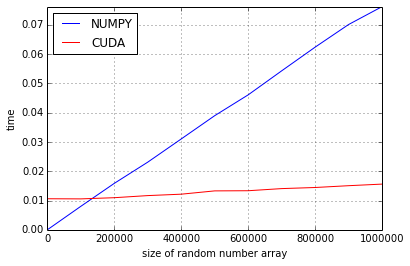The second test series with a pretty small workload.

In :
factor = 10
cudaTimes, cpuTimes = timeComparsion(factor)

Bytes of largest array 800080



The overhead of using the GPU is too large for small workloads.

In :
plot_results(cpuTimes, cudaTimes, factor)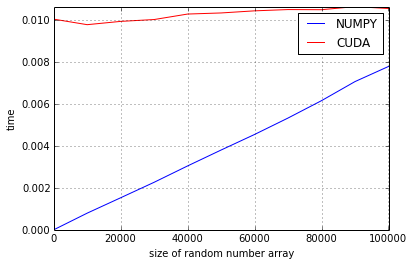Now a test series with heavy workloads.

In :
%%time
factor = 5000
cudaTimes, cpuTimes = timeComparsion(factor)

Bytes of largest array 400040000
CPU times: user 21 s, sys: 780 ms, total: 21.8 s
Wall time: 21.8 s



In :
plot_results(cpuTimes, cudaTimes, factor)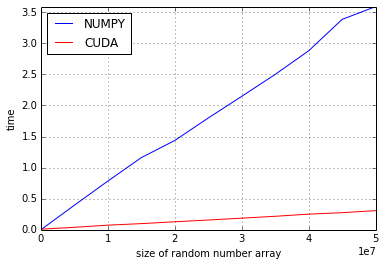## Distributed Execution of Python Functions¶

The algorithm we (arbitrarily) choose is the Monte Carlo estimator for a European option value in the Black-Scholes-Merton set-up. In this set-up the underlying follows the stochastic differential equation:

$dS_t = r S_t dt + \sigma S_t dZ_t$

The Monte Carlo estimator for a call option value looks like follows:

$C_0 = e^{-rT} \frac{1}{I} \sum_{i=1}^{I} \max[S_T(i) - K, 0]$

where $S_T(i)$ is the $i$-th simulated value of the underlying at maturity $T$.

A dynamic implementation of the BSM Monte Carlo valuation could look like follows.

In :
def BSM_MCS(S0):
''' Dynamic Black-Scholes-Merton MCS Estimator for European Calls. '''
import numpy as np
K = 100.; T = 1.0; r = 0.05; vola = 0.2
M = 50; I = 50000
dt = T / M
rand = np.random.standard_normal((M + 1, I))
S = np.zeros((M + 1, I)); S = S0
for t in range(1, M + 1):
S[t] = S[t-1] * np.exp((r - 0.5 * vola ** 2) * dt + vola * np.sqrt(dt) * rand[t])
option_value = np.sum(np.maximum(S[-1] - K, 0)) / I
return option_value


### The Sequential Calculation¶

The benchmark case of sequential valuation of $n$ options.

In :
def seqValue(n):
optionValues = []
for S in linspace(80, 100, n):
optionValues.append(BSM_MCS(S))
return optionValues


### The Parallel Calculation¶

First, start a (local) cluster.

In :
# in the shell ...
####
# ipcluster start -n 16
####
# or maybe more cores


Second, enable parallel computing capabilities.

In :
from IPython.parallel import Client
c = Client(profile="default")


The function for the parallel execution of a number $n$ of different option valuations.

In :
def parValue(n):
optionValues = []
for S in linspace(80, 100, n):
value = view.apply_async(BSM_MCS, S)
# asynchronously calculate the option values
optionValues.append(value)
c.wait(optionValues)
return optionValues


For a systematic performance comparison, we use the following funcion.

In :
def perf_comp_data(func_list, data_list, rep=3, number=1):
from timeit import repeat
res_list = {}
for name in enumerate(func_list):
stmt = name + '(' + data_list[name] + ')'
setup = "from __main__ import " + name + ', ' + data_list[name]
results = repeat(stmt=stmt, setup=setup, repeat=rep, number=number)
res_list[name] = sum(results) / rep
res_sort = sorted(res_list.iteritems(), key=lambda (k, v): (v, k))
for item in res_sort:
rel = item / res_sort
print 'function: ' + item + ', av. time sec: %9.5f, ' % item \
+ 'relative: %6.1f' % rel


Let us compare performance of the two different approaches.

In :
n = 20  # number of option valuations
func_list = ['seqValue', 'parValue']
data_list = 2 * ['n']

In :
perf_comp_data(func_list, data_list, rep=3)

function: parValue, av. time sec:   0.78208, relative:    1.0
function: seqValue, av. time sec:   5.73408, relative:    7.3



Performance scales (almost) linearly with the number of CPU cores available.

## Dynamic Compiling of Python Code using LLVM¶

The simulation of the movement of N bodies in relation to each other, where $N$ is a large number and a body can be a star/planet or a small particle, is an important problem in Physics.

The basic simulation algorithm involves a number of iterations ("nested loops") since one has to determine the force that every single body exerts on all the other bodies in the system to be simulated (e.g. the gravitational forces in place in a galaxy of billions of stars or the electro-magnetic forces in a system of atomic particles).

The simulation algorithm lends iteself pretty well for parallization. However, the example code that follows does not use any parallelization techniques. It illustrates rather what speed-ups are achievable by

• using vectorization approaches with NumPy and
• dynamic compiling capabilities of Numba

A description and discussion of the algorithm is found in the recent article Vladimirov, Andrey and Vadim Karpusenko (2013): "Test-Driving Intel Xeon-Phi Coprocessors with a Basic N-Body Simulation." White Paper, Colfax International.

### Pure Python¶

We first use pure Python to implement the algorithm. We start by generating random vectors of particle locations and a zero vector for the speed of each particle. All data is stored in lists of lists.

In :
import random
import math

In :
N = 150
M = 15
dt = 0.1

In :
p_py = []
for i in range(N):
p_py.append([random.gauss(0.0, 1.0) for j in range(3)])

In :
pv_py = [[0, 0, 0] for x in p_py]


Next, we define the simulation function for the movements of each particle given their (gravitational/electro-magnetic) interaction. The algorithm uses a number normalizations and simplifications which seems acceptable against the background that we only want to compare different versions of the same algorithm. In other words, we do not have to really care, for example, about the quality of the results, we shall rather focus on the efficiency with which the results are generated.

In :
def nbody_py(particle, particlev, M, N, dt):
''' Function to simulate the movement of N bodies.

Parameters
==========
particle : list of list objects
the position of the bodies in 3d space
particlev : list of list objects
the speed vectors of the bodies in 3d space
M: int
number of time steps to be simulated

Returns
=======
particle : list of list objects
the position of the bodies in 3d space
particlev : list of list objects
the speed vectors of the bodies in 3d space
AFTER SIMULATION
'''
for step in range(1, M + 1, 1):
for i in range(N):
Fx = 0.0; Fy = 0.0; Fz = 0.0
for j in range(N):
if j != i:
dx = particle[j] - particle[i]
dy = particle[j] - particle[i]
dz = particle[j] - particle[i]
drSquared = dx * dx + dy * dy + dz * dz
drPowerN32 = 1.0 / (drSquared + math.sqrt(drSquared))
Fx += dx * drPowerN32
Fy += dy * drPowerN32
Fz += dz * drPowerN32
particlev[i] += dt * Fx
particlev[i] += dt * Fy
particlev[i] += dt * Fz
for i in range(N):
particle[i] += particlev[i] * dt
particle[i] += particlev[i] * dt
particle[i] += particlev[i] * dt
return particle, particlev


We then execute the function to measure execution speed.

In :
%time p_py, pv_py = nbody_py(p_py, pv_py, M, N, dt)

CPU times: user 466 ms, sys: 5 ms, total: 471 ms
Wall time: 464 ms



### NumPy Solution¶

The basic algorithm allows for a number of vectorizations by using NumPy. However, we first need to generate our random sample data as a basis for the simulation. This time as NumPy array objects.

In :
import numpy as np

In :
p_np = np.random.standard_normal((N, 3))
pv_np = np.zeros_like(p_np)

Using NumP ndarray objects and matplotlib's 3d plotting capabilities, we can easily **visualize the intial positions** of the bodies in the space.
In :
import matplotlib.pyplot as plt
from mpl_toolkits.mplot3d import Axes3D

In :
fig = plt.figure()
ax.scatter(p_np[:, 0], p_np[:, 1], p_np[:, 2],
c='r', marker='.')

Out:
<mpl_toolkits.mplot3d.art3d.Patch3DCollection at 0x1289a1d0>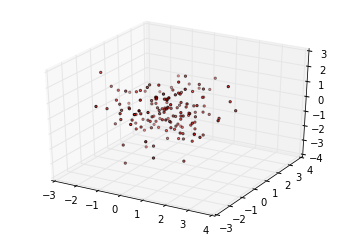The respective NumPy simulation function is considerably more compact due to the vectorization.

In :
def nbody_np(particle, particlev, M, N, dt):
''' Function to simulate the movement of N bodies (NumPy).

Parameters
==========
particle, particlev : NumPy ndarray objects
M : int

Returns
=======
particle, particlev : NumPy ndarray objects
'''
for step in range(1, M + 1, 1):
Fp = np.zeros((N, 3))
for i in range(N):
dp = particle - particle[i]
drSquared = np.sum(dp ** 2, axis=1)
h = drSquared + np.sqrt(drSquared)
drPowerN32 = 1. / np.maximum(h, 1E-10)
Fp += -(dp.T * drPowerN32).T
particlev += dt * Fp
particle += particlev * dt
return particle, particlev


It is also considerably faster.

In :
%time p_np, pv_np = nbody_np(p_np, pv_np, M, N, dt)

CPU times: user 119 ms, sys: 0 ns, total: 119 ms
Wall time: 118 ms



Let us check how the bodies moved. The following is a snapshot of the final positions of all bodies.

In :
fig = plt.figure()
ax.scatter(p_np[:, 0], p_np[:, 1], p_np[:, 2],
c='r', marker='.')

Out:
<mpl_toolkits.mplot3d.art3d.Patch3DCollection at 0x12a36250>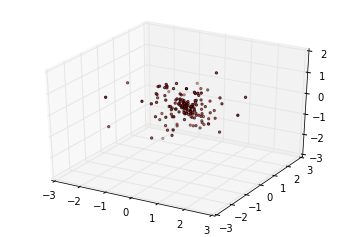### Dynamically Compiled Version¶

For this case, we first implement a function which is somehow a hybrid function of the pure Python (loop-heavy) and the NumPy array class-based version.

In :
def nbody_hy(particle, particlev, M, N, dt):
''' Function to simulate the movement of N bodies (for Numba).

Parameters
==========
particle, particlev : NumPy ndarray objects
M : int

Returns
=======
particle, particlev : NumPy ndarray objects
'''
for step in range(1, M + 1, 1):
for i in range(N):
Fx = 0.0; Fy = 0.0; Fz = 0.0
for j in range(N):
if j != i:
dx = particle[j, 0] - particle[i, 0]
dy = particle[j, 1] - particle[i, 1]
dz = particle[j, 2] - particle[i, 2]
drSquared = dx * dx + dy * dy + dz * dz
drPowerN32 = 1.0 / (drSquared + math.sqrt(drSquared))
Fx += dx * drPowerN32
Fy += dy * drPowerN32
Fz += dz * drPowerN32
particlev[i, 0] += dt * Fx
particlev[i, 1] += dt * Fy
particlev[i, 2] += dt * Fz
for i in range(N):
particle[i, 0] += particlev[i, 0] * dt
particle[i, 1] += particlev[i, 1] * dt
particle[i, 2] += particlev[i, 2] * dt
return particle, particlev


This function is then compiled with Numba.

In :
import numba as nb

In :
nbody_nb = nb.autojit(nbody_hy)


This new, compiled version of the hybrid function is yet faster.

In :
p_nb = np.random.standard_normal((N, 3))
pv_nb = np.zeros_like(p_nb)

In :
%timeit nbody_nb(p_nb, pv_nb, M, N, dt)

1 loops, best of 3: 10.2 ms per loop



### Speed Comparisons¶

The dynamically compiled version is by far the fastest.

In :
func_list = ['nbody_py', 'nbody_np', 'nbody_nb', 'nbody_hy']
data_list = ['p_py, pv_py, M, N, dt', 'p_np, pv_np, M, N, dt',
'p_nb, pv_nb, M, N, dt', 'p_nb, pv_nb, M, N, dt']

In :
perf_comp_data(func_list, data_list, rep=3)

function: nbody_nb, av. time sec:   0.01028, relative:    1.0
function: nbody_np, av. time sec:   0.11654, relative:   11.3
function: nbody_py, av. time sec:   0.46015, relative:   44.7
function: nbody_hy, av. time sec:   2.53499, relative:  246.5



### A Larger Example¶

We now use a larger set of bodies which we want to simulate. This illustrates that the Numba version of the algorithm is capable of shouldering quite a heavy workload.

In :
M = 50
N = 5000
dt = 1.0

In :
p_nb = np.random.standard_normal((N, 3)) * 1000
pv_nb = np.zeros_like(p_nb)

The following figure shows the much larger set of bodies visualized.
In :
fig = plt.figure()
ax.scatter(p_nb[:, 0], p_nb[:, 1], p_nb[:, 2],
c='r', marker='.')

Out:
<mpl_toolkits.mplot3d.art3d.Patch3DCollection at 0x12e54f90>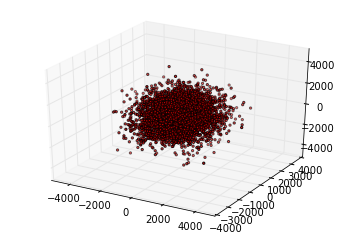Now the calculation with the Numba version.

In :
p_nb[:2]

Out:
array([[   69.27751998,  -315.02771294,   702.83000179],
[-1771.20470283, -1036.01381013, -1430.75681844]])

In :
%time p_nb, pv_nb = nbody_nb(p_nb, pv_nb, M, N, dt)

CPU times: user 35 s, sys: 0 ns, total: 35 s
Wall time: 35 s


In :
p_nb[:2]

Out:
array([[   6.79146685,  -84.40187229,   98.92071251],
[ 118.60133259,   57.47274369,   66.74096589]])

The result is shown graphically in the following figure.
In :
fig = plt.figure()
ax.scatter(p_nb[:, 0], p_nb[:, 1], p_nb[:, 2],
c='r', marker='.')

Out:
<mpl_toolkits.mplot3d.art3d.Patch3DCollection at 0x1315fd10>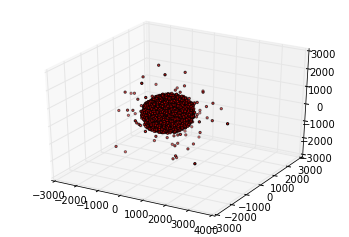The algorithmic example in this appendix shows which speed differences can be observed depending on different implementation approaches. Using Numba for dynamic compiling of Python functions might help in even improving significantly upon the speed of vectorized NumPy versions of the same algorithm.

## Why Python for (Big) Data Analytics & Visualization?¶

10 years ago, Python was considered exotic in the analytics space – at best. Languages/packages like R and Matlab dominated the scene. Today, Python has become a major force in data analytics & visualization due to a number of characteristics:

• multi-purpose: prototyping, development, production, sytems administration – Python is one for all
• libraries: there is a library for almost any task or problem you face
• efficiency: Python speeds up all IT development tasks for analytics applications and reduces maintenance costs
• performance: Python has evolved from a scripting language to a 'meta' language with bridges to all high performance environments (e.g. LLVM, multi-core CPUs, GPUs, clusters)
• interoperalbility: Python seamlessly integrates with almost any other language and technology
• interactivity: Python allows domain experts to get closer to their business and financial data pools and to do real-time analytics
• collaboration: solutions like Wakari with IPython Notebook allow the easy sharing of code, data, results, graphics, etc.## The Python Quants GmbH¶

The Python Quants – the company Web site

www.pythonquants.com

Dr. Yves J. Hilpisch – my personal Web site

www.hilpisch.com

Derivatives Analytics with Python – my new book

Read an Excerpt and Order the Book